Request a call back

# ICSE Class 10 Chemistry Previous Year Question Paper Semester 1 2021

Chemistry is an essential subject in ICSE Class 10, as the fundamentals studied now will also be helpful in competitive exams like IIT-JEE and NEET. The ICSE Class 10 Chemistry syllabus includes concepts on Periodic tables, Chemical bonding, Study of acids, bases and salts, metallurgy, organic chemistry, and many more. Each of these concepts is very important for higher grades.

TopperLearning presents study materials for ICSE Class 10 Chemistry to help students score well in the examination. All our study materials are created by Experienced and qualified subject experts and give fundamental insight on how and what to prepare for the final exams.

ICSE Class 10 Test section is recommended for students as it consists of MCQs and SAQs types of questions, which will help boost the practice required for related exams.

All the content resources are created by experienced and qualified faculty members who have handled students with their studies and aided in clarifying their academic doubts through the ‘UnDoubt’ platform. Get access to these crucial student-centric resources through ICSE Class 10 Plans.

Question 1

In the periodic Table, elements of period 3 are arranged in the increasing order of ionization potential as:               

1. B, N, Cl, Ar
2. Mg, Si, S, Ar
3. Ar, Si, S, Mg
4. Si, Ar, Cl, Mg

Question 2

If Relative Molecular Mass of Butane (C4H10) is 58 then its vapour density will be:                                                

1. (a)58
2. (b)29
3. (c)32
4. (d)16

Question 3

Identify one statement that holds true for electrolysis of molten lead bromide:                                                       

1. Silver grey metal deposits at the anode
2. Temperature is not maintained during the electrolysis
3. Brown vapours of bromine are obtained at the anode.
4. Electrolyte contains H+ ions along with Pb2+ ions

Question 4

The tendency of an atom to attract shared pair of electrons to itself when forming a chemical bond is known as:         

1. Electron affinity
2. Electronegativity
3. Ionization potential
4. Nuclear charge

Question 5

Solid sodium chloride does not conduct electricity as:                                                                                         

1. The strength of the bond is weak
2. It contains free ions
3. It does not contain any free ions
4. It contains free ions as well as molecules

Question 6

Elements A and B have electronic configurations 8 and 13 respectively. The chemical formula formed between A and B will be:

1. AB
2. B3A3
3. A2B3
4. B2A3

Question 7

The percentage of hydrogen present in NaOH is: (Relative Molecular Mass of NaOH = 40) (At. Wt. of H = 1)         

1. 2.5
2. 25
3. 0.25
4. 0.025

Question 8

A salt formed by incomplete neutralization of an acid by a base:                                                                         

1. Basic salt
2. Acid salt
3. Normal salt
4. Complex salt

Question 9

The colour of the precipitate formed after the addition of a small amount of sodium hydroxide solution to an aqueous solution of ferric chloride is:                                                                                                                                                                  

1. gelatinous white
2. pale blue
3. reddish brown
4. dirty green

Question 10

Alkaline earth metals have the same:                                                                                                                   

1. number of valence electrons
2. number of shells
3. metallic property
4. ionization potential

Question 11

Which of the following compounds neither dissociate not ionise in water?                                                           

1. Hydrochloric acid
2. Sodium hydroxide
3. Potassium Nitrate
4. Carbon tetrachloride

Question 12
The table shows the electronic configuration of four elements.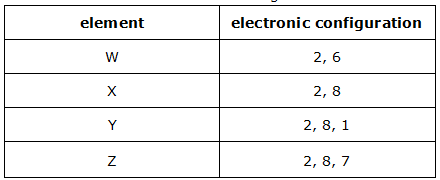Which pair of atoms will form a covalent compound?
1. two atoms of W
2. two atoms of X
3. an atom of W and an atom of X
4. an atom of Y and an atom of Z

Question 13
Element with an atomic number 19 will:                                                                                                             
1. accept an electron and get oxidized
2. accept an electron and get reduced
3. lose an electron and get oxidized
4. lose an electron and get reduced

Question 14
Which of the following has two sets of lone pair of electrons in them?                                                                    
1. Ammonia
2. Methane
3. Water
4. Ammonium ion

Question 15
If the empirical mass of the formula PQ2 is 10 and the Relative Molecular Mass is 30, then the molecular formula will be:               
1. PQ2
2. P3Q2
3. P6Q2
4. P3Q6

Question 16
Which of the following is a tribasic acid?                                                                                                      
1. H2SO4
2. Al(OH)3
3. H3PO4
4. Ca(OH)2

Question 17
If a solution of an electrolyte mixture has calcium ions, cupric ions, zinc ions and magnesium ions, which of these ions would you see preferentially discharged at the cathode?                                                                                                                
1. Calcium ions
2. Zinc ions
3. Cupric ions
4. Magnesium ions

Question 18
Which of the following ions will readily discharge at the anode during the electrolysis of acidulated water?                      
1. OH
2. SO42-
3. Cl-
4. H+

Question 19
If the imperial formula of a compound is CH and its vapour density is 13, then its molecular formula will be:             
(At. Wt. C=12, H=1)
1. CH
2. C2H2
3. C4H4
4. C3H3

Question 20
Aqueous solution of Cupric chloride forms a deep blue solution on addition of:                                                   
1. dropwise sodium hydroxide
2. excess sodium hydroxide
3. dropwise ammonium hydroxide
4. excess ammonium hydroxide

Question 21
Which statement about conduction of electricity is correct?                                                                                   
1. Electricity is conducted in aqueous solution by electrons
2. Electricity is conducted in a metal wire by ions
3. Electricity is conducted in a molten electrolyte by electrons
4. Electricity is conducted in an acid solution by ions

Question 22
If an element has low ionization potential, then it is likely to be a:                                                                          
1. metal
2. metalloid
3. non metal
4. inert gas

Question 23
Which electron arrangement for the outer shell electrons in a covalent compound is correct?
1.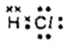2.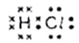3.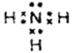4.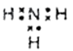Question 24

The products formed when an acid reacts with a base is:                                                                

1. salt and hydrogen
2. salt and oxygen
3. salt and water
4. salt and carbon dioxide

Question 25

In the circuit below, the lamp lights up.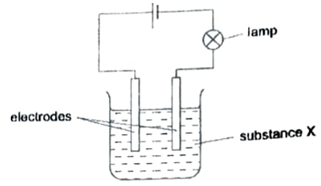What could X be?
1. a solution of alcohol in water
2. a solution of sodium chloride in water
3. sugar solution
4. solid potassium chloride

Question 26
Which one of the following is a non-metallic cation?                                                                                                
1. K+
2. NH4+
3. Cu2+
4. Na+

Question 27
Type of bonding present in hydrogen chloride:                                                                                                       
1. metallic
2. ionic
3. covalent
4. coordinate

Question 28
The non-metallic properties of elements from left to right in a Periodic Table:                                                           
1. increases
2. decreases
3. remains same
4. first increases and then decreases

Question 29
The aqueous solution that contains both ions and molecules:                                                                                   
1. sulphuric acid
2. nitric acid
3. acetic acid
4. hydrochloric acid

Question 30
The basic oxide which is an alkali:                                                                                                                           
1. Copper oxide
2. Sodium oxide
3. Ferric oxide
4. Zinc oxide

Question 31
If the pH of a solution is ‘2’, then solution is a:                                                                                                          
1. strong acid
2. strong alkali
3. weak acid
4. weak alkali

Question 32
The acidity of aluminium hydroxide is:                                                                                                                      
1. 3
2. 1
3. 4
4. 2

Question 33
Hydracids are those acids which contain:                                                                                                                     
1. Hydrogen with any metal
2. Hydrogen, a non-metal and oxygen
3. Hydrogen and a non-metal other than oxygen
4. Hydrogen and oxygen only

Question 34
The oxidation reaction among the following is:                                                                                                               
1. Fe3+ + 3e- → Fe
2. Fe2+ - Ie- → Fe3+
3. Cl2 + 2e- → 2Cl1-
4. Cu2+ + 2e- → Cu

Question 35
A student added excess of sodium hydroxide solution to each of the salt solution. An insoluble precipitate formed was observed in:         
1. Calcium nitrate
2. Zinc nitrate
4. Sodium nitrate

Question 36
Which apparatus could be used to electroplate an iron nail with copper?                                                                            
1.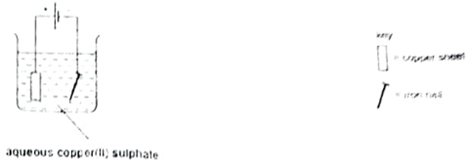2.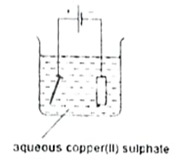3.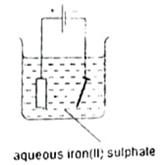4.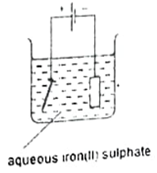Question 37

The table below shows the electronic arrangements of six atoms, A to F.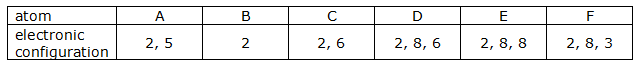With respect to the table select the following:

(i)Two atoms from the same group of the periodic table:                                                                                   

1. D and E
2. C and D
3. E and F
4. C and E

(ii)Two noble gases:                                                                                                                                     

1. A and B
2. E and F
3. B and E
4. D and E

(iii)The atom which is the most electronegative:                                                                                             

1. A
2. B
3. C
4. F

(iv)The atom which has the highest ionization potential:                                                                                      

1. A
2. B
3. E
4. F

## Key Features of Study Materials for ICSE Class 10 Chemistry:

• According to the latest ICSE syllabus
• Prepared by subject matter experts